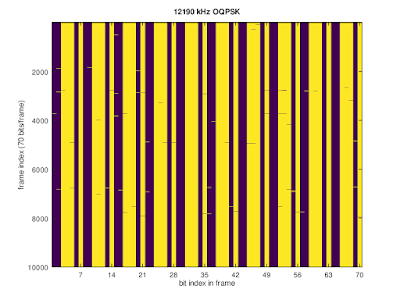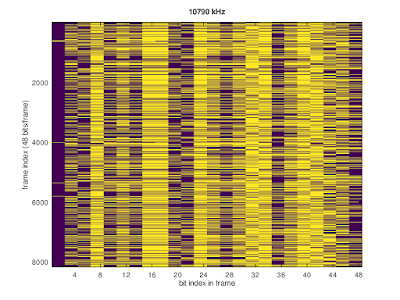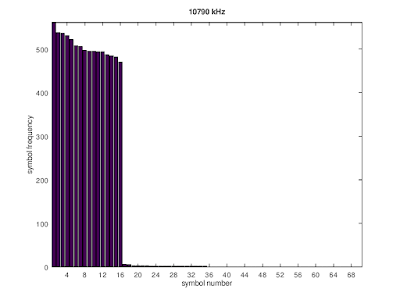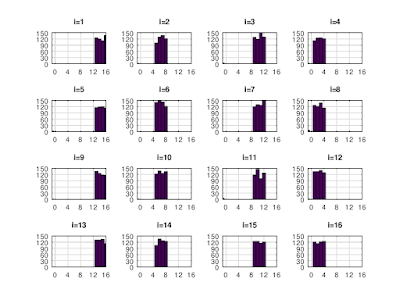## Monday, November 4, 2019

### Update on the OQPSK signal from Chicago

This is an update to this and to this blog post.

(Updated 11/5/19)

While before the offset between the "X" and "Y" bit streams was 458,748 bits, recently this offset has changed (but see below) to 65,536=216 bits, i.e., a non-pseudo-random bit stream can be obtained as follows:

m = 65536;
b = bitxor(bY(1:end-m),~bX(1+m:end)); # (*)

Recently this signal was observed on 12190 kHz with quite a lot of selective fading, so the coherent demodulation is not perfect.12190 kHz QQPSK demodulation

The bit stream obtained by using (*) shows a repeated pattern of 7 bits (0011101):fixed bit patten

It can be easily verified that this bit pattern is generated by an LFSR:
bn = bn-1 + bn-3 ,   (**)
where "+" denotes XOR. In fact the offset is the same as before. The fact that a LFSR-generated sequence is left after (**) is an artifact of using 1/7 original offset. This also makes the additional descrambling mentioned below obsolete.

At another time the same type of signal was observed on 10790 kHz, this time carrying data. Again the offset between the "X" and the "Y" bit streams was 216 bits. Using the LFSR (**) the resulting bit stream obtained from (*) can be descrambled, and then closely resembles the one found before: there are "frames" of 48-bits and the bits come in pairs, so in one given frames there are 24 "independent" (but see below) bits.bit stream in terms of 48-bit frames

Treating each line in the above plot as one "symbol" it was found that 16 symbols occur with (about equally) high frequency and all other symbols with very low frequency:48-bit symbol frequency
For now let's treat the symbols with low frequency as transmission errors (they might not be that).

The 16 symbols, when arranged in order, show an interesting pattern: in each group of four symbols 1) the last group of three bits is repeating, and 2) the first 7 groups of three bits are the same. In the table below the last number in brackets indicates the number of times a given symbol has occurred.

i= 1: 000_100_000_000_000_100_001_000 (497)
i= 2: 000_100_000_000_000_100_001_010 (494)
i= 3: 000_100_000_000_000_100_001_100 (531)
i= 4: 000_100_000_000_000_100_001_111 (487)

i= 5: 000_101_011_011_000_111_111_000 (470)
i= 6: 000_101_011_011_000_111_111_010 (561)
i= 7: 000_101_011_011_000_111_111_100 (536)
i= 8: 000_101_011_011_000_111_111_111 (506)

i= 9: 001_100_011_101_101_010_011_000 (494)
i=10: 001_100_011_101_101_010_011_010 (522)
i=11: 001_100_011_101_101_010_011_100 (495)
i=12: 001_100_011_101_101_010_011_111 (538)

i=13: 010_011_111_001_111_110_110_000 (508)
i=14: 010_011_111_001_111_110_110_010 (495)
i=15: 010_011_111_001_111_110_110_100 (485)
i=16: 010_011_111_001_111_110_110_111 (482)

The sequence of these symbols is not entirely random: the last three bits determine if the following symbol is in the group 13-16, 9-12, 5-8, or, 1-4:Distributions of symbols following the one indicated in the subplot title.

This might indicate a form of Trellis coding, because not all possible transitions between symbols are allowed.